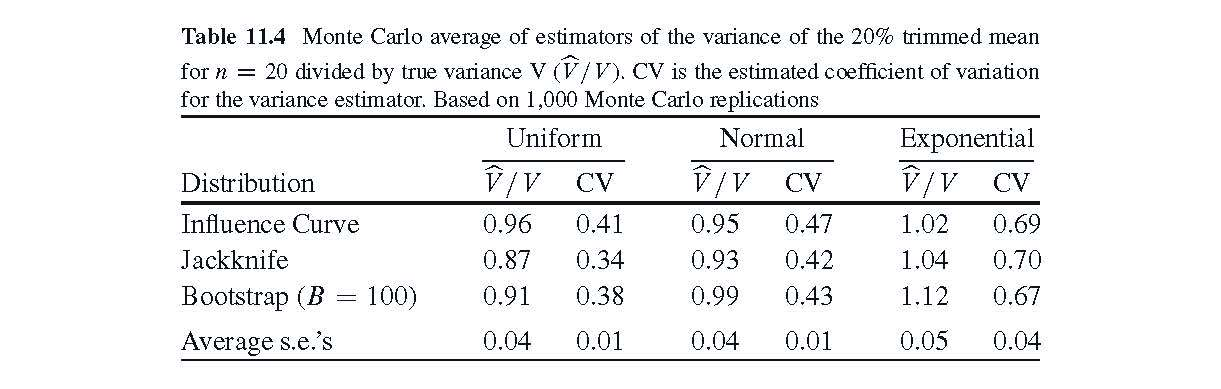# Monte Carlo Standard Errors for Pairwise Comparisons

#### 2019-05-01

In this vignette, we extend the analysis in Example 2 of Table 11.4, p. 423, of Boos and Stefanski (2013), “Essential Statistical Inference.” Table 11.4 compares three methods of estimating the variance of the 20% trimmed mean. The three methods are the Influence Curve Method (Delta Method), the jackknife, and the bootstrap. The main entries of the table are based on N Monte Carlo samples of size n=20, leading to a matrix of three sets, one set for each distribution: the N 20% trimmed means and 3 variance estimators. Here we focus on the 3 columns of Table 11.4 based on

CV = the coefficient of variation of the N variance estimatorsUnder the uniform distribution, the estimated CVs are 0.41, 0.34, and 0.38. With an approximate SE of 0.01, these three CVs are reasonably well-separated (“significantly different”). However, for the other two distributions, the CVs are not as well-separeated compared to their SEs. In such a case, one might be interested in the standard errors of the differences or of the ratios of the CVs, because the these latter standard errors should be less than if the summaries were independent. The function pairwise.se is designed to get all pairwise SEs.

Here we display the first line of the function:

pairwise.se <- function(x,xcol,diff=TRUE,digits=4,B=0,seed=NULL,summary.f,...){

We see the main arguments are the Monte Carlo raw output data x, the columns xcol of x to use, and the summary summary.f. Here, summary.f is just a simple function of one vector as used in mc.se.vector. In the example below, we use summary.f=CV.

In the Simulation Code below, we generate an N=1,000 by 12 matrix hold of Monte Carlo outout. Columns 1-4 of hold are for the uniform(0,1) distribution, columns 5-8 are for the standard normal distribution, and columns 9-12 are for the standard exponential distribution. Columns 1, 4, and 8 are 20% trimmed means, and the other columns are estimated variances.

To illustrate pairwise.se, we first get the standard errors of the differences of CV values in Table 11.4 for the normal distribution using columns 6-8 of the output data frame hold.

> pairwise.se(hold,xcol=6:8,summary.f=cv)
elem  summi  summj summary     se  t.stat    N    method
1  6 7 0.4695 0.4232  0.0463 0.0072  6.4254 1000 Jackknife
2  6 8 0.4695 0.4359  0.0337 0.0090  3.7556 1000 Jackknife
3  7 8 0.4232 0.4359 -0.0126 0.0071 -1.7795 1000 Jackknife

First notice that the summaries (0.47, 0.42, 0.43) for the normal distribution in Table 11.4
show up under summi and summj. Then their differences are listed under summary. The standard errors of the differences are under se. Next, t.stat is summary/se if differences are used and (summary-1)/se if ratios are used (diff=F). To illustrate the use of ratios,

> pairwise.se(hold,xcol=6:8,diff=F,summary.f=cv)
elem  summi  summj summary     se  t.stat    N    method
1  6 7 0.4695 0.4232  1.1095 0.0178  6.1676 1000 Jackknife
2  6 8 0.4695 0.4359  1.0773 0.0216  3.5719 1000 Jackknife
3  7 8 0.4232 0.4359  0.9710 0.0160 -1.8174 1000 Jackknife

Notice that summi and summj are the same as for differences, but now summary holds the ratios. The t statistics are similar to those for differences.
If the bootstrap is to be used in place of the jackknife, we have

> pairwise.se(hold,xcol=6:8,B=1000,seed=769,summary.f=cv)
elem  summi  summj summary     se  t.stat    B seed    N    method
1  6 7 0.4695 0.4232  0.0463 0.0070  6.5859 1000  769 1000 Bootstrap
2  6 8 0.4695 0.4359  0.0337 0.0090  3.7520 1000  769 1000 Bootstrap
3  7 8 0.4232 0.4359 -0.0126 0.0068 -1.8640 1000  769 1000 Bootstrap

> pairwise.se(hold,xcol=6:8,diff=F,B=1000,seed=769,summary.f=cv)
elem  summi  summj summary     se  t.stat    B seed    N    method
1  6 7 0.4695 0.4232  1.1095 0.0178  6.1481 1000  769 1000 Bootstrap
2  6 8 0.4695 0.4359  1.0773 0.0211  3.6563 1000  769 1000 Bootstrap
3  7 8 0.4232 0.4359  0.9710 0.0160 -1.8179 1000  769 1000 Bootstrap

# Simulation Code

The following code creates an N=1,000 by 12 matrix ‘hold’ of Monte Carlo outout. Columns 1-4 are for the uniform(0,1) distribution, columns 5-8 are for the standard normal distribution, and columns 9-12 are for the standard exponential distribution. Columns 1, 4, and 8 are 20% trimmed means, and the other columns are estmated variances.

trim20 <- function(x){mean(x,.2)} # 20% trimmed mean function
trim.var <- function(x,trim){     # var. est. for trim20
n <- length(x);h<-floor(trim*n)
tm <- mean(x,trim);sort(x)->x
ss <- h*((x[h+1]-tm)^2+(x[n-h]-tm)^2)
ss <- ss+sum((x[(h+1):(n-h)]-tm)^2)
ss/((n-2*h)*(n-2*h-1))
}
trim20.var<-function(x){trim.var(x,.2)}

N=1000
hold <- matrix(0,nrow=N,ncol=12)

set.seed(351)
z <- matrix(runif(N*20),nrow=N)
hold[,1] <- apply(z,1,trim20)
hold[,2] <- apply(z,1,trim20.var)
hold[,3] <- apply(z,1,jack.var,theta=trim20)
hold[,4] <- apply(z,1,boot.var,theta=trim20,B=100)

set.seed(352)
z<- matrix(rnorm(N*20),nrow=N)
hold[,5] <- apply(z,1,trim20)
hold[,6] <- apply(z,1,trim20.var)
hold[,7] <- apply(z,1,jack.var,theta=trim20)
hold[,8] <- apply(z,1,boot.var,theta=trim20,B=100)

set.seed(353)
z<- matrix(rexp(N*20),nrow=N)
hold[,9] <- apply(z,1,trim20)
hold[,10] <- apply(z,1,trim20.var)
hold[,11] <- apply(z,1,jack.var,theta=trim20)
hold[,12] <- apply(z,1,boot.var,theta=trim20,B=100)